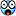calculating the value of pi - Page 3

# Thread: calculating the value of pi

1.Registered User
Join Date
Mar 2001
Posts
729
One question I have though.. How is the computer calculating the trig functions? I like the sum one because I know what it is doing.2.Registered User
Join Date
Apr 2001
Location
SF Bay Area, CA
Posts
14,947
The x86 math coprocessors (x87 if x <= 3) have instructions that can be used to calculate trig functions.

I don't know how accurate (or precise) they are, though... probably only to the machine floating-point precision (either 32 or 64 bits, or 80 if your compiler supports "long double").

3. ## the computer isn't...

with the atan suggestions, the taylor series expansion is used...
atan(x) = x - x^3/3 + x^5/5 - x^7/7 ...
or
atan(x) = summation from n=1 to infinity of (-1)^(n+1)*x^(2n-1) / (2n-1)
so each iteration you just add another term and it gets closer to the exact value of atan(x), which can be used to find pi in a number of ways.

4. Are you sure the computer uses Taylor series? They're very slow; I think x86s have some binary method which involves mathematical rotations.

5. sorry, misunderstanding. no, i highly doubt that the computer uses the taylor series. i'm saying the programmer could use that method to get perfect accuracy for atan, instead of using the machine's approximation of atan.

6.Registered User
Join Date
Jul 2003
Posts
28

7. Originally posted by o0zi
Why calculate when you know it to several places from memory?3.141592635358979323846264338327950288419716939937

(yes, that was from memory)
Damn! That is three digits more than I have memorized!

8. just use the pi button on a calculator.

ha ha.

9. ##Million Dollar que\$tion

Why?

Why do you need to calculate pi down so far?

Are you perchance shooting a rocket across the galaxy and need to hit a target on Vega the size of a grapefruit or what?(That'd be really cool if you were though)10. You might as well ask the same of most of mathematics - it's an intellectual pursuit which many people enjoy merely for the fun of it, and not the practical value.

11.Musketeer
Join Date
Aug 2003
Location
Auburn, WA
Posts
83
Originally posted by o0zi
Why calculate when you know it to several places from memory?3.141592635358979323846264338327950288419716939937

(yes, that was from memory)
Don't know if this was a typo or not, but a published value is:

3.141592653589793238462643383279502884197169399375 11

(you've got an extra 3 in the 8th digit to the right of the decimal point).12. Yup, sorry, typo3.141592653589793238462643383279502884197169399375 10582

13.Registered User
Join Date
Mar 2001
Posts
729
You just put that there to make us think you took it from memory.

Impostor.14. Originally posted by drummerboy195
we are working on sums of sequences in clac right now, and if it wasnt for the pesky change in signs every other number, it could be expressed as the sum of 4(1/(2i-1)) as i goes from 1 to infinity. except, the real sum should be 4(1/(2i-1)-1/(2i/1)+1/(2i-1)-1/(2i/1)+1/(2i-1)........) anybody know how to set that up?
4*sum((-1)^(i+1)/(2*i-1),i,1,infinity)=4(1-1/3+1/5-1/7...)

15. ## Re: Million Dollar que\$tion

Originally posted by knute
Why?
That's how nerds entertain themselves.

Andrew Wiles locked himself up in an attic for 7 years working up a proof to Fermat's Last Theorem. Nobody knows what to do with it now, but at least Wiles had fun.####Posting Permissions

• You may not post new threads
• You may not post replies
• You may not post attachments
• You may not edit your posts
•